# Bloch theorem

Bloch theorem is a fundamental result in the theory of solids that describes the behaviour of electrons in a periodic potential, such as the crystal lattice of a solid. The theorem is named after the Swiss physicist Felix Bloch, who first published it in 1928.

According to the Bloch theorem, the wave function of an electron in a periodic potential can be written as the product of a periodic function and a plane wave. The periodic function describes the behavior of the electron within a unit cell of the crystal lattice, while the plane wave describes its propagation through the lattice.

The periodic function is known as the Bloch wave function, and it satisfies certain boundary conditions at the boundaries of the unit cell. These boundary conditions ensure that the wave function remains continuous and differentiable throughout the crystal lattice.

The Bloch theorem has important consequences for the electronic structure of solids. It predicts the existence of energy bands in the electronic structure, which are separated by band gaps. The width of these bands and gaps depends on the properties of the crystal lattice and the nature of the interactions between the electrons.

The Bloch theorem has been used extensively in the study of semiconductors, metals, and insulators, and it provides a powerful framework for understanding the behaviour of electrons in condensed matter systems.

In case of free electron theory in 1 D Schrodinger equation for an electron moving in a constant potential Vo is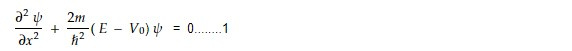Solution of this equation are plane waves of the type

For an electron moving in 1- D periodic potential V(x), Schrodinger equation is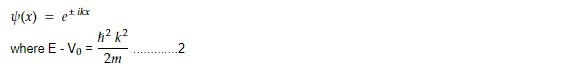let a = period of periodic potential

V(x) = V (x+a)

According to Bloch theorem, equation can be modulated by a function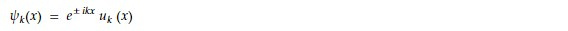Number of wavefunction in a band

Let L be length of a linear crystal using the periodic boundary condition

Ψ ( x + L) = Ψ (x)

This boundary condition implies that the wavefunction has the same value at x and x+L, which means that the wavefunction is periodic with a period of L.

In the context of solid-state physics, this periodic boundary condition can be used to model the behaviour of electrons in a crystalline material. The wavefunction of an electron in a crystal can be thought of as a periodic function that describes the electron's behaviour in the crystal lattice. The periodic boundary condition ensures that the wavefunction is consistent across the boundaries of the crystal.

For Bloch wavefunction,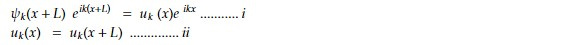From equation i and ii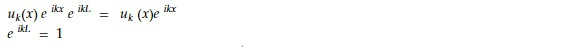= 2πn/ L .......iii

From iii, n = kL/ 2π

The number of wave functions in the interval dk is

dn = L dk/2π

Total number of possible states in a band is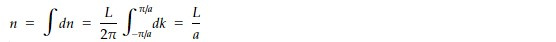or, na = L

For first Brillouin zone, k max = π /a

N-> Number of primitive cells in crystal of length L

a = L/ N

Maximum value of k in first Brillouin zone is Nπ / L. Thus the series, equation iv is cut off at Nπ / L = Nπ / Na = π /a. This is zone boundary.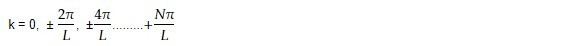[-Nπ / L = - π /a is not counted as an independent point because it is connected by a reciprocal lattice vector with π /a] . The total number of primitive cells is N. Hence the total number of possible states in any energy band is equal to number of primitive cells.

This note is a part of the Physics Repository.